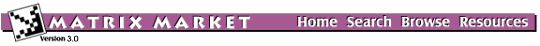## MVMRWK: Markov Chain Transition Matrix

### from the NEP Collection

Matrix Generator MVMRWK
Source: G.W. Stewart, University of Maryland
Discipline: Probability theory and its applications
Language: Fortran
Output format: matrix-vector multiply

Consider a random walk on an (m+1) × (m+1) triangular grid, illustrated below for m = 6.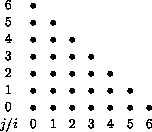The points of the grid are labeled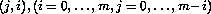. From the point (j,i), a transition may take place to one of the four adjacent points (j+1,i), (j,i+1), (j-1,i), (j,i-1). The probability of jumping to either of the nodes (j-1,i) or (j,i-1) is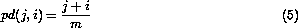with the probability being split equally between the two nodes when both nodes are on the grid. The probability of jumping to either of the nodes (j+1,i) or (j,i+1) is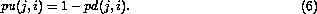with the probability again being split when both nodes are on the grid. If the (m+1)(m+2)/2 nodes (j,i) are numbered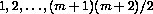in some fashion, then the random walk can be expressed as a finite Markov chain with transition matrix A of order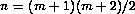consisting of the probabilities akl of jumping from node l to node k (A is actually the transpose of the usual transition matrix; see [Feller]).

We are primarily interested in the steady state probabilities of the chain, which is ordinarily the appropriately scaled eigenvector corresponding to the eigenvalue unity.

Figure 1 shows the sparsity pattern of the resulted random walk matrix of order 136 (i.e. m=15).

To calculate the i-th element of the vector Ax one need only regard the components of q as the average number of individuals at the nodes of the grid and use the probabilities (5) and (6) to calculate how many individuals will be at node i after the next transition.

Parameters:

 N order of the matrix. Must me (M+1)(M+2)/2 for some positive M.